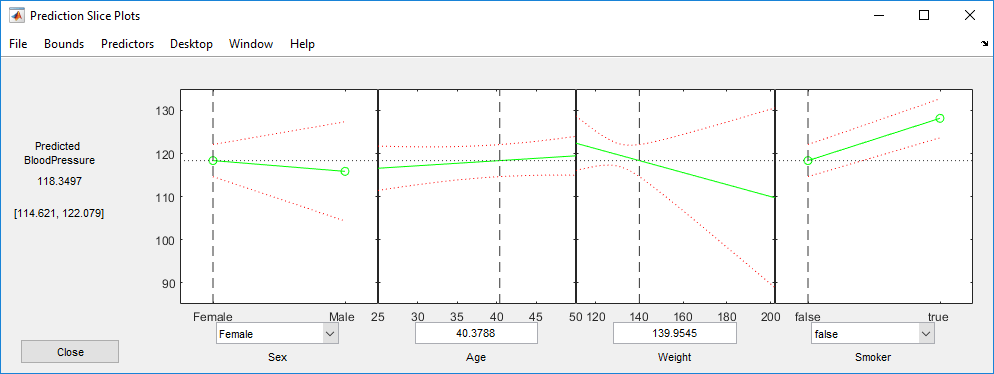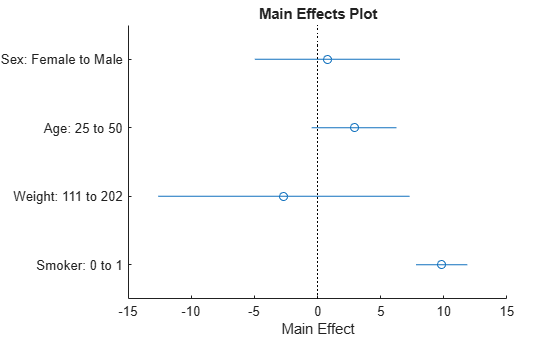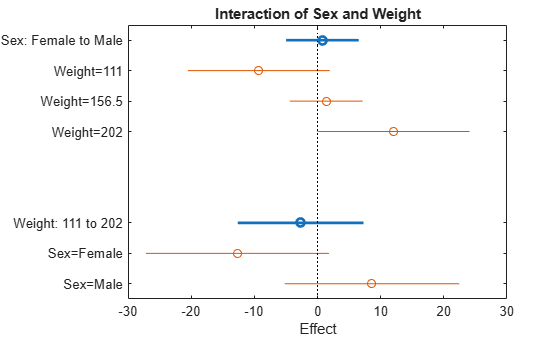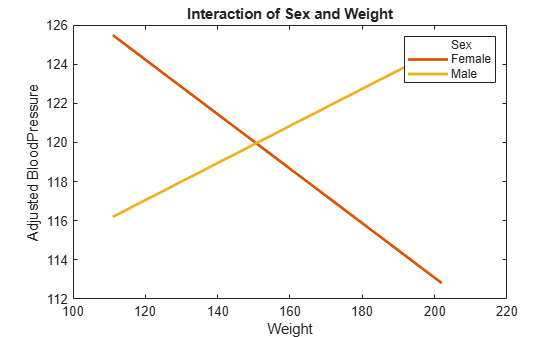Main Content

# Linear Regression with Interaction Effects

Construct and analyze a linear regression model with interaction effects and interpret the results.

### Load sample data.

`load hospital`

To retain only the first column of blood pressure, store data in a table.

```tbl = table(hospital.Sex,hospital.Age,hospital.Weight,hospital.Smoker,hospital.BloodPressure(:,1), ... 'VariableNames',{'Sex','Age','Weight','Smoker','BloodPressure'});```

### Perform stepwise linear regression.

For the initial model, use the full model with all terms and their pairwise interactions.

`mdl = stepwiselm(tbl,'interactions')`
```1. Removing Sex:Smoker, FStat = 0.050738, pValue = 0.8223 2. Removing Weight:Smoker, FStat = 0.07758, pValue = 0.78124 3. Removing Age:Weight, FStat = 1.9717, pValue = 0.16367 4. Removing Sex:Age, FStat = 0.32389, pValue = 0.57067 5. Removing Age:Smoker, FStat = 2.4939, pValue = 0.11768 ```
```mdl = Linear regression model: BloodPressure ~ 1 + Age + Smoker + Sex*Weight Estimated Coefficients: Estimate SE tStat pValue ________ ________ _______ __________ (Intercept) 133.17 10.337 12.883 1.76e-22 Sex_Male -35.269 17.524 -2.0126 0.047015 Age 0.11584 0.067664 1.712 0.090198 Weight -0.1393 0.080211 -1.7367 0.085722 Smoker_1 9.8307 1.0229 9.6102 1.2391e-15 Sex_Male:Weight 0.2341 0.11192 2.0917 0.039162 Number of observations: 100, Error degrees of freedom: 94 Root Mean Squared Error: 4.72 R-squared: 0.53, Adjusted R-Squared: 0.505 F-statistic vs. constant model: 21.2, p-value = 4e-14 ```

The final model in formula form is `BloodPressure ~ 1 + Age + Smoker + Sex*Weight`. This model includes all four main effects (Age, Smoker, Sex, Weight) and the two-way interaction between `Sex` and `Weight`. This model corresponds to

`$BP={\beta }_{0}+{\beta }_{A}{X}_{A}+{\beta }_{Sm}{I}_{Sm}+{\beta }_{S}{I}_{S}+{\beta }_{W}{X}_{W}+{\beta }_{SW}{X}_{W}{I}_{S}+ϵ,$`

where

• $BP$ is the blood pressure

• ${\beta }_{i}$ are the coefficients

• ${I}_{Sm}$ is the indicator variable for smoking; ${I}_{Sm}=1$ indicates a smoking patient whereas ${I}_{Sm}=0$ indicates a nonsmoking patient

• ${I}_{S}$ is the indicator variable for sex; ${I}_{S}=1$ indicates a male patient whereas ${I}_{S}=0$ indicates a female patient

• ${X}_{A}$ is the `Age` variable

• ${X}_{W}$ is the `Weight` variable

• $ϵ$ is the error term

The following table shows the fitted linear model for each gender and smoking combination.

As seen from these models, ${\beta }_{Sm}$ and ${\beta }_{S}$ show how much the intercept of the response function changes when the indicator variable takes the value 1 compared to when it takes the value 0. ${\beta }_{SW}$, however, shows the effect of the Weight variable on the response variable when the indicator variable for sex takes the value 1 compared to when it takes the value 0. You can explore the main and interaction effects in the final model using the methods of the `LinearModel` class as follows.

### Plot prediction slice plots.

```figure plotSlice(mdl)```This plot shows the main effects for all predictor variables. The green line in each panel shows the change in the response variable as a function of the predictor variable when all other predictor variables are held constant. For example, for a smoking male patient aged 37.5, the expected blood pressure increases as the weight of the patient increases, given all else the same.

The dashed red curves in each panel show the 95% confidence bounds for the predicted response values.

The horizontal dashed line in each panel shows the predicted response for the specific value of the predictor variable corresponding to the vertical dashed line. You can drag these lines to get the predicted response values at other predictor values, as shown next.For example, the predicted value of the response variable is 118.3497 when a patient is female, nonsmoking, age 40.3788, and weighs 139.9545 pounds. The values in the square brackets, [114.621, 122.079], show the lower and upper limits of a 95% confidence interval for the estimated response. Note that, for a nonsmoking female patient, the expected blood pressure decreases as the weight increases, given all else is held constant.

### Plot main effects.

`plotEffects(mdl)`This plot displays the main effects. The circles show the magnitude of the effect and the blue lines show the upper and lower confidence limits for the main effect. For example, being a smoker increases the expected blood pressure by 10 units, compared to being a nonsmoker, given all else is held constant. Expected blood pressure increases about two units for males compared to females, again, given other predictors held constant. An increase in age from 25 to 50 causes an expected increase of 4 units, whereas a change in weight from 111 to 202 causes about a 4-unit decrease in the expected blood pressure, given all else held constant.

### Plot interaction effects.

```figure plotInteraction(mdl,'Sex','Weight')```This plot displays the impact of a change in one factor given the other factor is fixed at a value.

Be cautious while interpreting the interaction effects. When there is not enough data on all factor combinations or the data is highly correlated, it might be difficult to determine the interaction effect of changing one factor while keeping the other fixed. In such cases, the estimated interaction effect is an extrapolation from the data.

The blue circles show the main effect of a specific term, as in the main effects plot. The red circles show the impact of a change in one term for fixed values of the other term. For example, in the bottom half of this plot, the red circles show the impact of a weight change in female and male patients, separately. You can see that an increase in a female’s weight from 111 to 202 pounds causes about a 14-unit decrease in the expected blood pressure, while an increase of the same amount in the weight of a male patient causes about a 5-unit increase in the expected blood pressure, again given other predictors are held constant.

### Plot prediction effects.

```figure plotInteraction(mdl,'Sex','Weight','predictions')```This plot shows the effect of changing one variable as the other predictor variable is held constant. In this example, the last figure shows the response variable, blood pressure, as a function of weight, when the variable sex is fixed at males and females. The lines for males and females are crossing which indicates a strong interaction between weight and sex. You can see that the expected blood pressure increases as the weight of a male patient increases, but decreases as the weight of a female patient increases.

Download ebook